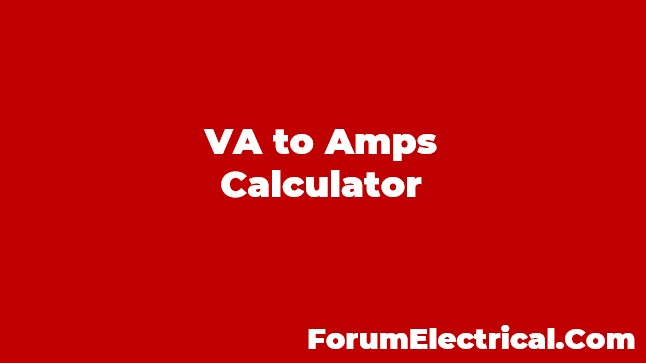# VA to Amps Calculator

0
295The apparent power, measured in volt-amps (VA), can be converted to the current, measured in amps, using the VA to Amps Calculator (A). Both the single phase and the three phase are available on this calculator.

## Formula for converting single phase volt-amperes to amps:

I = VA / V

I, measured in amps, is equivalent to the apparent power S, measured in volt-amps, divided by the voltage V, measured in volts.

## Formula for converting three phase volt-amperes to amps:

I = VA / √3 × VL-L = VA / 3 × VL-N

I in amps is equal to the apparent power S in volt-amps, and this value is then divided by the square root of three times the voltage from line to line, which is measured in volts.

Enter phase, volt-amps, and voltage: